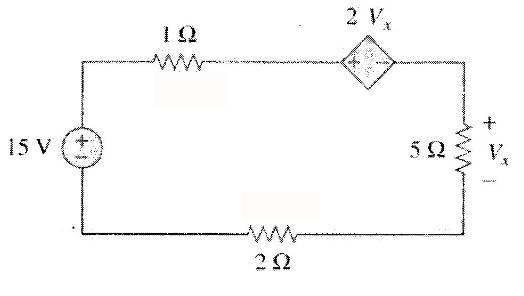# Find the Voltage in the following circuit diagram

• Engineering
• vaio911
In summary, the student is trying to find V_x in a circuit but is having trouble solving for it. He is using some equations to help, but is unsure if he needs the resistance values V1 and V2. The circuit he is working with generates current, which is the same throughout the circuit. Lastly, he is unsure if the diamond source he is working with is a voltage or current controlled source.f

## Homework Statement

Find $V_x$ in the circuit.## Homework Equations

$\displaystyle{\sum V_i=0}$

## The Attempt at a Solution

I tried to add all of the voltages and label the 2 ohm res V1 and 1 ohm res V2.

Then I did:

$V_x+V_1-15+V_2+2V_x=0$

$3V_x+V_1+V_2=15$

but then I couldn't find any other equations that will aid me in solving this.
I'm not really sure if I really need the V1 and V2.
The circuit doesn't give you the current either, so I don't know why resistance would help.

Thanks.

## Homework Statement

Find $V_x$ in the circuit.## Homework Equations

$\displaystyle{\sum V_i=0}$

## The Attempt at a Solution

I tried to add all of the voltages and label the 2 ohm res V1 and 1 ohm res V2.

Then I did:

$V_x+V_1-15+V_2+2V_x=0$

$3V_x+V_1+V_2=15$

but then I couldn't find any other equations that will aid me in solving this.
I'm not really sure if I really need the V1 and V2.
The circuit doesn't give you the current either, so I don't know why resistance would help.

Thanks.

Welcome to the PF.

Where did you come up with those equations? There is one current around that loop that generates the voltage drops and controls the voltage controlled current source. Could you please try writing the one equation for the loop again, and solving it?

Is this right?

$i$ is the current

\begin{align*} 2V_x+V_x+2i+i&=15\\ 3(5\cdot i)+2i+i&=15\\ i&=\frac{15}{18}=\frac{5}{6}\;A \end{align*}

$V=I\cdot R=\frac56 \cdot 5=\boxed{\dfrac{25}{6}\;V}$

Also, current is the same throughout in a series circuit right/

Is this right?

$i$ is the current

\begin{align*} 2V_x+V_x+2i+i&=15\\ 3(5\cdot i)+2i+i&=15\\ i&=\frac{15}{18}=\frac{5}{6}\;A \end{align*}

$V=I\cdot R=\frac56 \cdot 5=\boxed{\dfrac{25}{6}\;V}$

The diamond source is a voltage controlled current source, right? Not a voltage...

Also, current is the same throughout in a series circuit right/

Correct.

Upon further review, and input from another Mentor, I may be wrong about the controlled source. It looks like a current source to me, but I could be wrong about that. It may be a voltage controlled voltage source (VCVS) instead. Sorry if I mislead you on this.